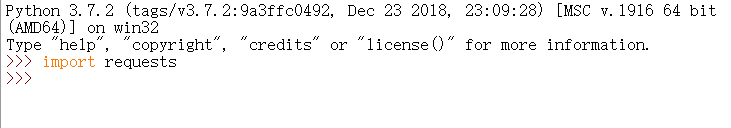``````#coding:utf-8

import requests
import sys
from time import time

def Usage():

def getHTML(URL):
html = get(URL).text
return html

def saveFile(Fname,Fdata):
f = open(Fname,'w')
f.write(Fdata)
f.close()

def getName():
name = str(time())
return name

def resetEC():
sys.setdefaultencoding('utf-8')

if __name__ == '__main__':
try:
URL = sys.argv
resetEC()
html = getHTML(URL)
name = getName()
saveFile(name+'txt',html)
print '[INFO]Save file success'
except:
Usage()
``````